This article contains a shorter technical description of the requirement and of the solution, and a longer explanation of the reasons why the solution proposed is required and why other approaches may fail. If you are familiar with calculation groups in DAX, you probably only need to read the first part of the article which is short and straight to the point. If calculation groups are relatively new to you, or if you would like to read a more detailed explanation about why this technique is required, then you can also enjoy the second part of the article. That longer section is designed to first make sense of the problem, then of the technique used, and possibly to make you want to know calculation groups better. Ready? Let’s begin!

## Part 1: Applying a calculation group to a specific measure

Calculation groups replace measure references with the DAX expression of the calculation item that is active in the filter context. Because the replacement takes place only at the report level where the calculation groups are usually applied, it is not possible to propagate the replacement to measure references used in nested calculations. This behavior limits your ability to apply calculation groups only to a specific subexpression of a complex DAX calculation.

For example, consider the following Daily Sales and Monthly Sales measures:

```Daily Sales :=
DIVIDE ( [Sales Forecast], [Days] )

Monthly Sales :=
DIVIDE ( [Sales Forecast], [Months] )
```

If your requirement is to modify the Sales Forecast measure through a slicer selection, you cannot use a calculation group to define different implementations of Sales Forecast. You should rewrite the DIVIDE expressions in the calculation group because SELECTEDMEASURE corresponds to Daily Sales or Monthly Sales.

The solution is to move the original Sales Forecast implementation into an Internal Sales Forecast measure; then, you want to define Sales Forecast with a measure that applies the required calculation item based on the current selection of a table (called Choice) that contains a copy of the items of the calculation group (called InternalChoice):

```Sales Forecast :=
CALCULATE (
[Internal Sales Forecast],
TREATAS (
VALUES ( Choice[Selection] ),
InternalChoice[Selection]
)
)
```

The Sales Forecast implementation controls the application of the calculation item so that the latter affects only the Internal Sales Forecast measure. The Choice table is the only one visible to the user, whereas the calculation group implemented as InternalChoice is not visible to the user.

This technique defers the application of the calculation group to the desired expressions; it does so without limiting your ability to create other measures on top of the ones affected by the calculation group.

## Part 2: Understanding how calculation groups can be applied to partial expressions

We know: Part 1 is hard. However, you do not need to be scared. Our goal was to introduce the problem and pique your interest so you would read this long article – you may need it in the future when faced with a similar scenario. You can read Part 1 again once you complete the article. At that point, you will be able to understand all the implications of this calculation technique.

Calculation groups require a lot of concentration. The first important detail we always need to remember with calculation groups is that things are seldom as easy as we would like them to be. Forgetting the theory of evaluation and application of calculation items can result in very undesired and hard-to-understand results. To demonstrate this, we start by analyzing a common scenario; we then guide you in understanding the problem, and finally we guide you towards the solution. We wrote the article describing exactly the path that led to the solution. In other words, we describe the same reasoning and the same mistakes we did back when we were studying the topic. This article is a sneak peek into two hours of real life at SQLBI!

In order to show the problem with the Contoso database, we assumed that Contoso sometimes analyzes their sales taking the discounts into account, and sometimes ignoring them. Therefore, the value of Sales Amount can be computed using the Unit Price – which is not reflecting any discounts – or using the Net Price, consequently taking the discount as part of the equation.

Different values for Sales Amount have a domino effect on other measures. The margin percentage for example is different depending on whether you consider the Unit Price or the Net Price. If you look at the following figure, you can see that the margin computed using Net Price (Margin % NP) is lower than the margin considering the Unit Price (Margin % UP).In order to build the report, we have authored different versions of the measures which reflect the different ways of computing Sales Amount:

```Sales Amount (NP) :=
SUMX ( Sales, Sales[Quantity] * Sales[Net Price] )

Sales Amount (UP) :=
SUMX ( Sales, Sales[Quantity] * Sales[Unit Price] )

Margin % (NP) :=
VAR SalesAmount = Sales[Sales Amount (NP)]
VAR SalesCost = [Total Cost]
VAR Result =
DIVIDE ( SalesAmount - SalesCost, SalesAmount )
RETURN
Result

Margin % (UP) :=
VAR SalesAmount = Sales[Sales Amount (UP)]
VAR SalesCost = [Total Cost]
VAR Result =
DIVIDE ( SalesAmount - SalesCost, SalesAmount )
RETURN
Result
```

In a real model, the number of measures would quickly grow: you might end up having two versions of each measure, depending on the definition of Sales Amount. The problem is not limited to the measures. The reports should be duplicated too, because the algorithm is hardcoded in the measures used by the report. We need to find a better solution.

This looks like a perfect candidate for a calculation group. We define a new calculation group to let the user choose the price to use when computing Sales Amount and – thanks to some DAX magic – the entire report updates itself using the required version of Sales Amount. We want to produce a report with a PriceToUse slicer like in the following picture.We need a calculation group with two calculation items. When the user selects Net Price, we replace Sales Amount with the formula using Net Price. Alternatively, when the user selects Unit Price, we use the formula with Unit Price. The calculation item needs to replace the formula. Therefore, it does not use SELECTEDMEASURE; it merely replaces the entire expression:

```CALCULATIONITEM 'PriceToUse'[PriceToUse]."Net Price" =
SUMX ( Sales, Sales[Quantity] * Sales[Net Price] )

CALCULATIONITEM 'PriceToUse'[PriceToUse]."Unit Price" =
SUMX ( Sales, Sales[Quantity] * Sales[Unit Price] )
```

For Margin %, we rely on the calculation item to perform its magic. Therefore, we just call a generic version of Sales Amount, trusting that the calculation item updates it accordingly:

```Margin % :=
VAR SalesAmount = [Sales Amount]
VAR SalesCost = [Total Cost]
VAR Result =
DIVIDE (
SalesAmount - SalesCost,
SalesAmount
)
RETURN
Result
```

When used in a report, this calculation group proves to be too invasive. By replacing the selected measure with the new formula, it ends up replacing the entire report with the value of Sales Amount, as shown in the following screenshot.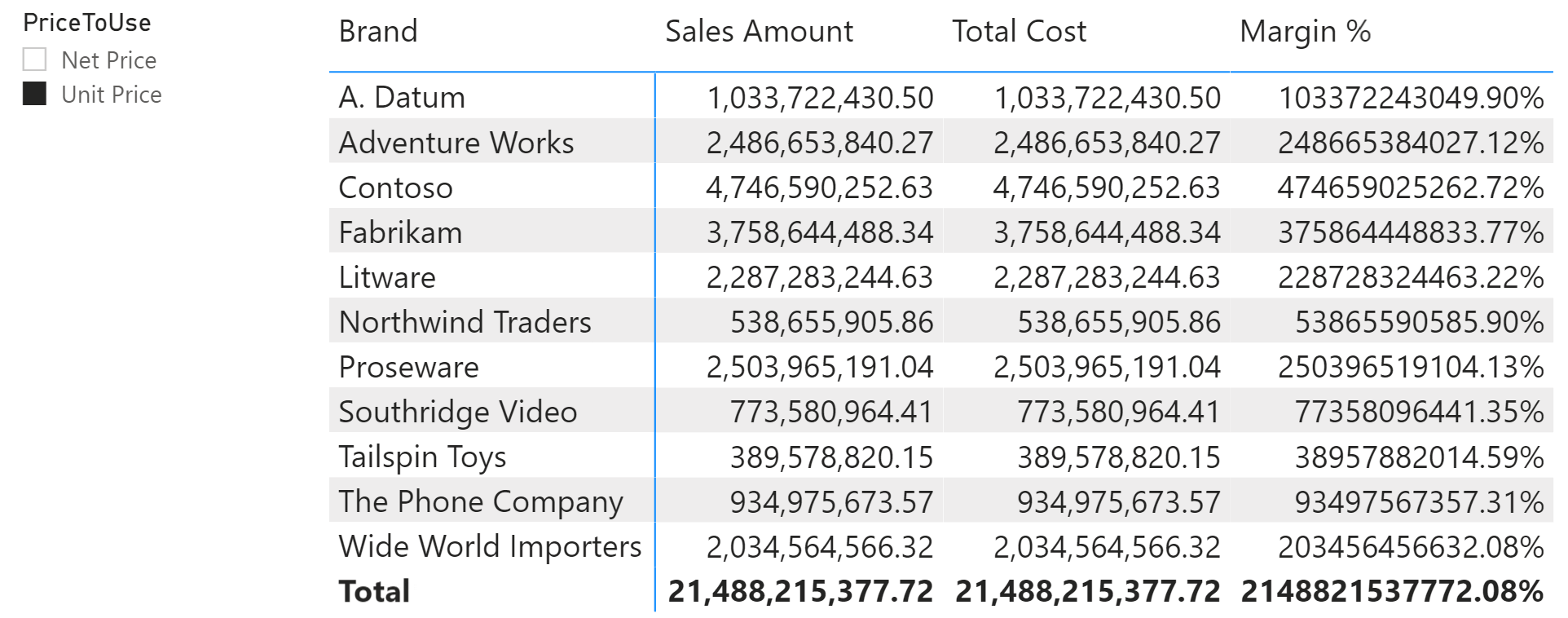Here is what is happening: the calculation item replaces the selected measure – whatever that may be – with the value of Sales Amount. Therefore, it does not matter whether the report references Sales Amount, Total Cost, or Margin %. All these measures are replaced with Sales Amount. As you can see from the report, the only detail remaining of the original measure is the format string.

We want to tell the calculation item that it needs to affect only Sales Amount, while keeping the other measures untouched. DAX offers the ISSELECTEDMEASURE function to check the name of the selected measure. It looks as though an IF statement is all we need:

```CALCULATIONITEM PriceToUse[PriceToUse]."Net Price" =
IF (
ISSELECTEDMEASURE ( [Sales Amount] ),
SUMX ( Sales, Sales[Quantity] * Sales[Net Price] )
)

CALCULATIONITEM PriceToUse[PriceToUse]."Unit Price" =
IF (
ISSELECTEDMEASURE ( [Sales Amount] ),
SUMX ( Sales, Sales[Quantity] * Sales[Unit Price] )
)
```

Unfortunately, this solution is still wrong: as you can see, it works with Sales Amount but it blanks out all the remaining measures.This is to be expected. During the execution of the IF functions, if any measure other than Sales Amount is the one selected then the else branch returns blank. As usual DAX is doing what we are asking it to do, and it is not doing what we want it to do… Regardless, it looks like we only need to fix the else branch of both IF functions by returning SELECTEDMEASURE. This way, it replaces only Sales Amount, and it should not replace any other measure:

```CALCULATIONITEM PriceToUse[PriceToUse]."Net Price" =
IF (
ISSELECTEDMEASURE ( [Sales Amount] ),
SUMX ( Sales, Sales[Quantity] * Sales[Net Price] ),
SELECTEDMEASURE ()
)

CALCULATIONITEM PriceToUse[PriceToUse]."Unit Price" =
IF (
ISSELECTEDMEASURE ( [Sales Amount] ),
SUMX ( Sales, Sales[Quantity] * Sales[Unit Price] ),
SELECTEDMEASURE ()
)
```

The result is still wrong. This time both the issue and the reason why it happens are not easy to understand. To make the issue more evident, we added into the report the measures that compute the margin with Unit Price and Net Price.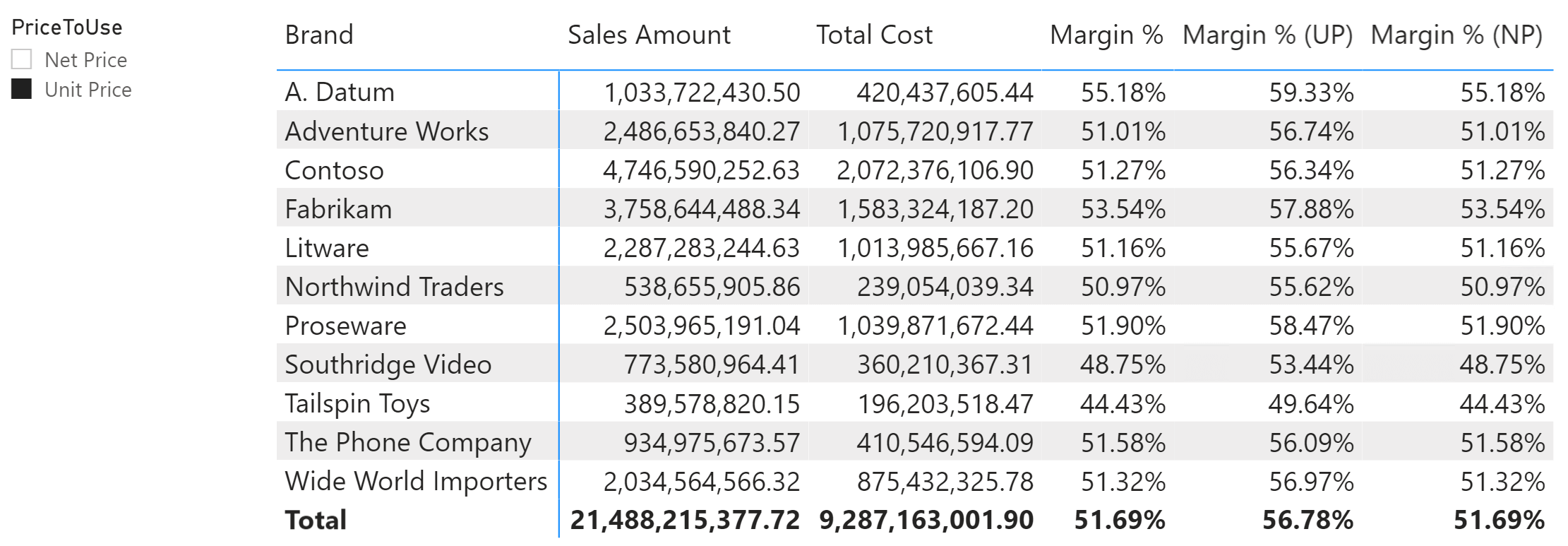Here is the problem: we selected Unit Price. Sales Amount is showing the correct value by using the Unit Price in its internal calculations. On the other hand, Margin % is equal to Margin % (NP), and they show the margin still by using the Net Price. For example, A.Datum should display 59.33% instead of 55.18% as Margin %.

To make sense of this, we need to understand what happens when the calculation item is applied. Power BI executes a query which requests the application of the Unit Price calculation item. The query – a bit simplified – looks like this:

```DEFINE
VAR __DS0FilterTable =
TREATAS ( { "Unit Price" }, 'PriceToUse'[PriceToUse] )
EVALUATE
SUMMARIZECOLUMNS (
'Product'[Brand],
__DS0FilterTable,
"Sales_Amount", 'Sales'[Sales Amount],
"Total_Cost", 'Sales'[Total Cost],
"Margin__", 'Sales'[Margin %]
)
```

The calculation item is applied to the three measures: Total Cost, Margin %, and Sales Amount. Each time, the corresponding measure is returned by SELECTEDMEASURE.

When the calculation item is applied to Sales Amount, it replaces Sales Amount with the correct version that computes the value using Unit Price. This is what we wanted.

When the calculation item is applied to Total Cost, it applies SELECTEDMEASURE which is the original measure: Total Cost. Still fine.

The issue is with Margin %. When the calculation item is applied to Margin %, the behavior is the very same as the one described for Total Cost. Because the SELECTEDMEASURE is not Sales Amount, the calculation item transforms the selected measure in the selected measure itself, not changing its result.

Once the calculation item is applied, the engine computes the code of Margin %. It does not matter that inside Margin % we are using Sales Amount. The calculation item is applied only once at the top-level calculation and never again. Once we have asked DAX not to do anything – because the selected measure is not Sales Amount – the application of the calculation item is completed. No further steps take place. Therefore, the internal reference to Sales Amount inside Margin % is not replaced with the calculation we want.

This is the important thing about calculation groups: when you define a calculation group and you protect it with an IF statement that checks the result of ISSELECTEDMEASURE, you are not telling the engine that the calculation item must be applied to only that measure. You are modifying the application of the calculation item to any measure, choosing different expansions based on which measure you are replacing. The reference to Sales Amount inside Margin % remains untouched. Because the default implementation of Sales Amount uses Net Price, Margin % is not changing its behavior based on the calculation item: it is always using the default version of the measure.

Understanding this last detail is of paramount importance. If the previous two paragraphs are not crystal clear, please take another tour of the calculation groups series of articles to refresh your understanding of the internals of calculation groups.

Now that we understand the problem, we still need to solve it. We need to force the calculation item to operate only on the Sales Amount measure, thus overriding the default behavior. In order to achieve this goal, we cannot let the user choose the calculation item. Indeed, if the calculation group is placed in the report, it is filtered straight in the query. As such, the application of the calculation group occurs at the top level of the calculation, where SELECTEDMEASURE is the measure being queried.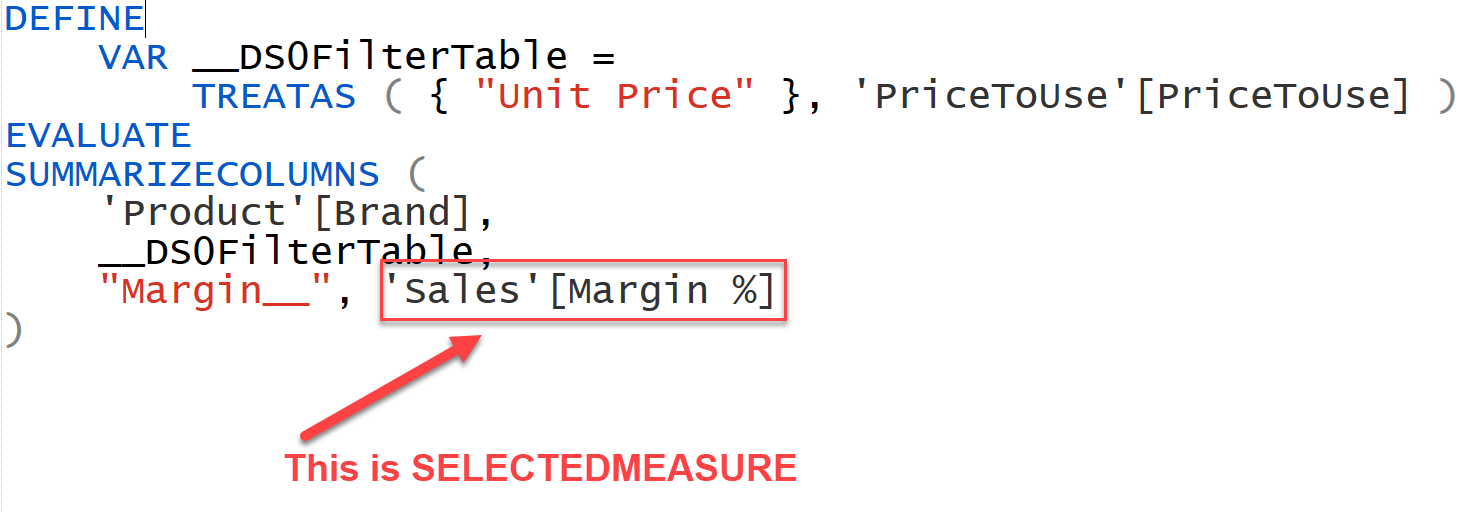What we want to happen is different. If we expand the definition of Margin % in the previous query, we can better understand where we want the calculation item to be applied: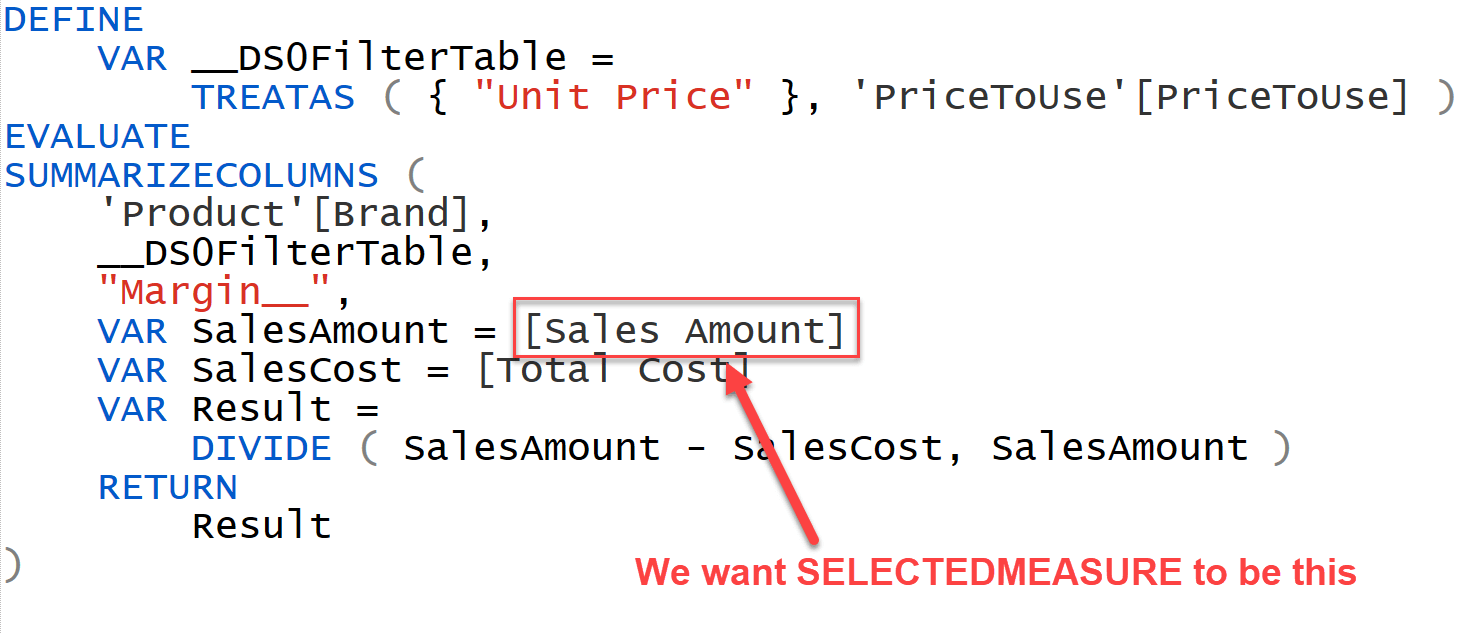We can force the application of a calculation item to a specific part of our calculation by using CALCULATE to filter the calculation group. This CALCULATE needs to read the desired value from a table accessible to the user.

Therefore, these are the steps required:

• Since we do not want the report to operate directly on the calculation group, we rename it as InternalPriceToUse and we hide it. This cannot guarantee that a user will not mess up the calculation… But it provides a smooth experience for non-malicious users.
• We create a parameter table PriceToUse disconnected from the model, with the same content as the calculation group. The user operates on this table through a slicer.
• We add CALCULATE around the [Sales Amount] measure reference. It reads the content of the disconnected table and uses it to force the application of the calculation item exactly where we want it to happen.

Here is an excerpt of the final model below.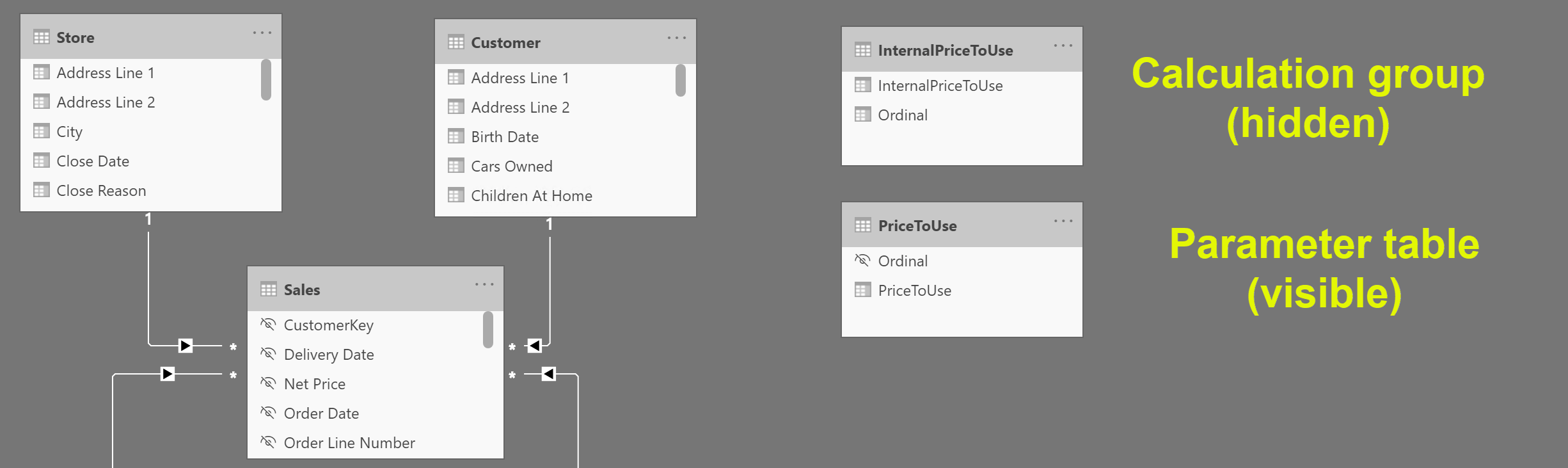The parameter table must have the same values as the internal calculation group. We can build it by using a simple DAX calculated table:

```PriceToUse =
SELECTCOLUMNS (
InternalPriceToUse,
"PriceToUse", InternalPriceToUse[InternalPriceToUse],
"Ordinal", InternalPriceToUse[Ordinal]
)
```

The last step requires adding CALCULATE around the Sales Amount reference. To achieve this, we create a new Internal Sales Amount hidden measure that returns the default value of Sales Amount. In the Sales Amount measure, we use CALCULATE to invoke the Internal Sales Amount measure by applying the required calculation item in the filter context. We choose the calculation item to use by reading the selected value in the parameter table:

```Internal Sales Amount :=
SUMX (
Sales,
Sales[Quantity] * Sales[Net Price]
)

SalesAmount :=
CALCULATE (
[Internal Sales Amount],
TREATAS (
VALUES ( PriceToUse[PriceToUse] ),
InternalPriceToUse[InternalPriceToUse]
)
)
```

By embedding CALCULATE in Sales Amount, there is no need to change the code of Margin %. Whenever a measure calls Sales Amount, the measure takes care of reading the required price to use from the parameter table and it applies the calculation item accordingly. The calculation item application does not take place in the query; it takes place in the measure. Exactly where we wanted it to take place.

Finally, there is no need to check what the selected measure is in the calculation items. Indeed, because we are using an internal calculation group, we have full control over when the calculation items are applied. As such, we can relax the safety of the code, and thus improve the performance:

```CALCULATIONITEM 'InternalPriceToUse'[PriceToUse]."Net Price" =
SUMX ( Sales, Sales[Quantity] * Sales[Net Price] )

CALCULATIONITEM 'InternalPriceToUse'[PriceToUse]."Unit Price" =
SUMX ( Sales, Sales[Quantity] * Sales[Unit Price] )
```

With all the required steps in place, now the user experience is what we wanted it to be. A slicer lets the user choose the price to use in the calculation of Sales Amount. Any measure using Sales Amount will modify its behavior and use the correct calculation.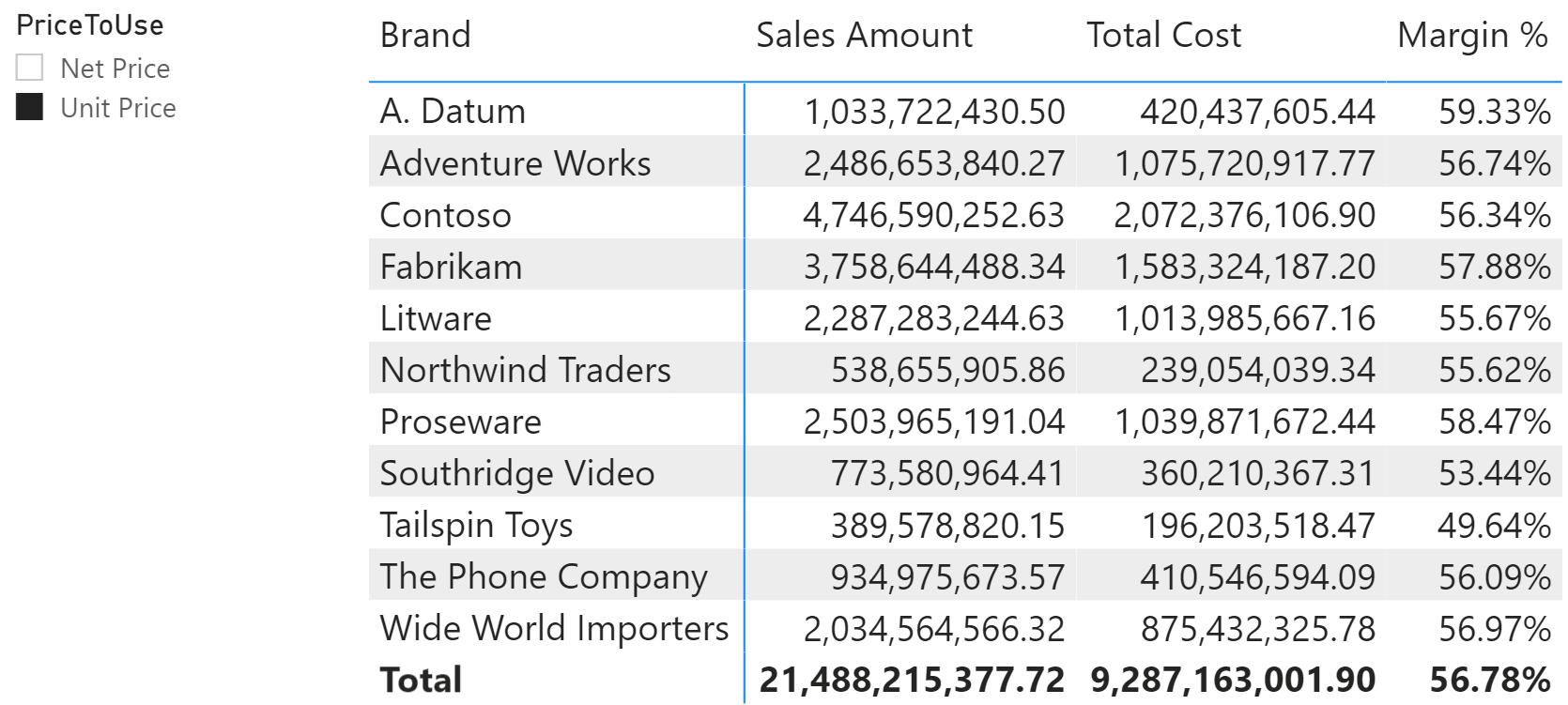You can consider this example as a pattern to inject any parameter from the report inside your measures, by using calculation groups instead of IF statements – as we were used to doing before the advent of calculation groups.

Indeed, we could solve the same scenario using SWITCH or IF statements without calculation groups at all. By using the calculation item, we move the definition of the expression to use into a different part of the model, which is easy to maintain and extend. By using a SWITCH or IF statement in Sales Amount we could implement a similar logic, but any change would affect the code in Sales Amount. The main motivation to use calculation groups is the maintainability of the solution. From a performance point of view, the results could be different and related to other details in the model. For larger and more complex models, the calculation groups approach could also provide better performance – although preparing a benchmark is always critical.

We will never grow tired of repeating that performance must be measured. Do not take for granted that a solution using calculation groups is faster than other alternatives. It might be, it might not. Your task as a DAX developer is to test multiple solutions and provide to your users the best one for each specific model. Have fun with DAX!

DIVIDESafe Divide function with ability to handle divide by zero case.

`DIVIDE ( <Numerator>, <Denominator> [, <AlternateResult>] )`

SELECTEDMEASUREReturns the measure that is currently being evaluated.

`SELECTEDMEASURE ( )`

ISSELECTEDMEASUREReturns true if one of the specified measures is currently being evaluated.

`ISSELECTEDMEASURE ( <Measure> [, <Measure> [, … ] ] )`

IFChecks whether a condition is met, and returns one value if TRUE, and another value if FALSE.

`IF ( <LogicalTest>, <ResultIfTrue> [, <ResultIfFalse>] )`

CALCULATEContext transition

Evaluates an expression in a context modified by filters.

`CALCULATE ( <Expression> [, <Filter> [, <Filter> [, … ] ] ] )`

SWITCHReturns different results depending on the value of an expression.

`SWITCH ( <Expression>, <Value>, <Result> [, <Value>, <Result> [, … ] ] [, <Else>] )`